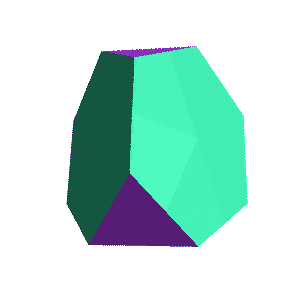Up: The Archimedean Solids

# The Tetrahedron and the Truncated TetrahedronTetrahedron 4 triangular faces4 verticesTruncated Tetrahedron 4 hexagonal faces,4 triangular faces

### What's happening?

To cut off the corners of the tetrahedron, we move in the same distance from each corner, along the edges. The distance we move is less than half of the side length, because it is impossible to move in more. (If we move in exactly half way before truncating, the result is the octahedron)

This process shortens the side lengths of the edges in the original tetrahedron by the same amount. Notice that it also doubles the number of edges -- changing the green triangular faces of the tetrahedron (left) into green hexagonal faces in the truncated tetrahedron (right).

In the tetrahedron, three faces meet at every vertex. Cutting off the original vertices, then, leaves a triangular shape behind, which is filled in by the purple triangles.

Next: Truncated Cube
Go back to The Archimedean Solids